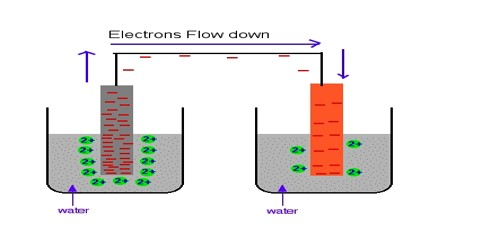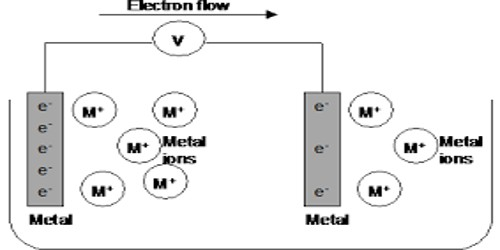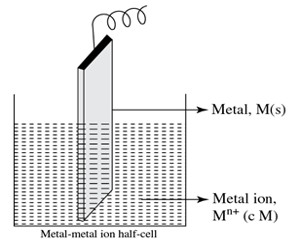# Metal-metal Ion Electrode in Half-Cells

Metal-metal ion electrode in Half-Cells

A half cell is one of the two electrodes in a galvanic cell or simple battery. For example, in the Zn-Cu battery, the two half cells make an oxidizing-reducing couple. In this case, the metal strip is kept in contact with the solution of a water-soluble salt-containing cation of the similar metal. e.g. Zn(s) | Zn++(aq)

Metal-metal ion electrode: This is the simplest type of electrode where metal is dipped into a solution of as ions. These electrodes consist of a pure metal (M) in contact with a solution of its cation (Mn+). For example, a silver rod immersed in a solution of Ag+ ions or copper rod in a copper sulphate solution. Examples are silver in silver nitrate solution, copper in a copper sulphate solution. The silver-silver ion electrode is represented as, Ag│Ag+ and the copper-copper ion electrode as Cu│Cu2+.The electrode reactions are Ag+ + e ↔ Ag and Cu2+ + 2e ↔ Cu respectively. The electrodes are reversible with respect to the metal ions. In the electrochemical cell, the electrode having higher oxidation potential undergoes oxidation and acts as the anode or negative electrode and the electrode having lower oxidation potential undergoes reduction and acts as the cathode or positive electrode.

In this type of half-cell, the metal rod is dipped in a solution of the corresponding metal ion having concentration c M.The half cell is shown in above Figure when functions as an anode can be represented as –

M(s) | Mn+ (c M)

The anodic half cell reaction is represented as –

M(s) → Mn+ (ag) + ne

The representation of the half cell when it functions as a cathode is: Mn+ (c M) | M(s).

The cathodic half cell reaction is Mn+(aq) + ne → M(s).

Examples of this kind of half cell, when used as cathode, are Cu2+ | Cu, Zn2+ Zn, Ag+ | Ag, Sn2+ | Sn, etc. The half cells or electrodes used in Daniel cell are of the metal-metal ion type.